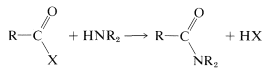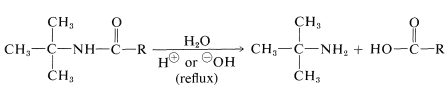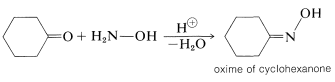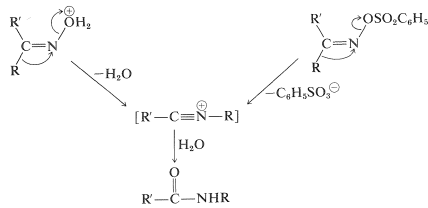# 24.3: Synthesis of Amides

$$\newcommand{\vecs}{\overset { \rightharpoonup} {\mathbf{#1}} }$$ $$\newcommand{\vecd}{\overset{-\!-\!\rightharpoonup}{\vphantom{a}\smash {#1}}}$$$$\newcommand{\id}{\mathrm{id}}$$ $$\newcommand{\Span}{\mathrm{span}}$$ $$\newcommand{\kernel}{\mathrm{null}\,}$$ $$\newcommand{\range}{\mathrm{range}\,}$$ $$\newcommand{\RealPart}{\mathrm{Re}}$$ $$\newcommand{\ImaginaryPart}{\mathrm{Im}}$$ $$\newcommand{\Argument}{\mathrm{Arg}}$$ $$\newcommand{\norm}{\| #1 \|}$$ $$\newcommand{\inner}{\langle #1, #2 \rangle}$$ $$\newcommand{\Span}{\mathrm{span}}$$ $$\newcommand{\id}{\mathrm{id}}$$ $$\newcommand{\Span}{\mathrm{span}}$$ $$\newcommand{\kernel}{\mathrm{null}\,}$$ $$\newcommand{\range}{\mathrm{range}\,}$$ $$\newcommand{\RealPart}{\mathrm{Re}}$$ $$\newcommand{\ImaginaryPart}{\mathrm{Im}}$$ $$\newcommand{\Argument}{\mathrm{Arg}}$$ $$\newcommand{\norm}{\| #1 \|}$$ $$\newcommand{\inner}{\langle #1, #2 \rangle}$$ $$\newcommand{\Span}{\mathrm{span}}$$

## From Carboxylic Acids

Formation of amides from carboxylic acid derivatives already has been discussed in some detail (Section 23-9A):$$\tag{24-1}$$

The ease of formation of amides by the reaction of Equation 24-1 depends a lot on the nature of the leaving group $$\ce{X}$$. The characteristics of a good leaving group were discussed in Sections 8-7C and 8-7D in connection with $$S_\text{N}$$ reactions, and similar considerations apply here. Some idea of the range of acid derivatives used in amide synthesis can be obtained from Table 24-1, which lists various $$\ce{RCOX}$$ compounds and the p$$K_a$$ values of $$\ce{HX}$$. As a reasonable rule of thumb, the stronger $$\ce{HX}$$ is as an acid, the better $$\ce{X}$$ is as a leaving group.

Table 24-1: Derivatives and Reactivity of Carboxylic Acids Commonly Used in Amide Formation

$\ce{RCOX} + \ce{H_2NR'} \rightarrow \ce{RCONHR'} + \ce{HX}$Amides generally are formed from acid chlorides, acid azides, acid anhydrides, and esters. It is not practical to prepare them directly from an amine and a carboxylic acid without strong heating or unless the reaction is coupled to a second reaction that "activates" the acid. Notice that esters of phenols are more reactive toward amines than esters of alcohols because phenols are stronger acids than alcohols.

## From Nitriles

The hydrolysis of nitriles is a satisfactory method for preparation of unsubstituted amides and is particularly convenient when hydrolysis is induced under mildly basic conditions by hydrogen peroxide:For the preparation of amides of the type $$\ce{R_3CNHCOR}$$, which have a tertiary alkyl group bonded to nitrogen, the Ritter reaction of an alcohol or alkene with a nitrile or hydrogen cyanide is highly advantageous. This reaction involves formation of a carbocation by action of strong sulfuric acid on an alkene or an alcohol (Equation 24-2), combination of the carbocation with the unshared electrons on nitrogen of $$\ce{RCN}$$ (Equation 24-3), and then addition of water (Equation 24-4). We use here the preparation of an $$\ce{N}$$-tert-butylalkanamide as an example; $$\ce{RC \equiv N}$$ can be an alkyl cyanide such as ethanenitrile or hydrogen cyanide itself:$$\tag{24-2}$$$$\tag{24-3}$$$$\tag{24-4}$$

This reaction also is useful for the preparation of primary amines by hydrolysis of the amide. It is one of the relatively few practical methods for synthesizing amines with a tertiary alkyl group on the nitrogen:## The Beckmann Rearrangement of Oximes

You may recall that ketones react with $$\ce{RNH_2}$$ compounds to give products with a double bond to nitrogen, $$\ce{-C=NR}$$ (Section 16-4C). When the $$\ce{RNH_2}$$ compound is azanol (hydroxylamine), $$\ce{HO-NH_2}$$, the product is called a ketoxime, or oxime:Oximes rearrange when heated with a strong acid, and this reaction provides a useful synthesis of amides:This intriguing reaction is known as the Beckmann rearrangement. It has been the subject of a number of mechanistic studies that have shown the acid or acid halide ($$\ce{PCl_3}$$, $$\ce{C_6H_5SO_2Cl}$$) makes the hydroxyl group on nitrogen into a better leaving group by forming $$\ce{-OH_2^+}$$ or ester intermediates:Thereafter, a rearrangement occurs resembling the reactions of carbocations (Sections 8-9B and 15-5E). When the cleavage of the $$\ce{N-O}$$ bond occurs, the nitrogen atom would be left with only six valence electrons. However, as the bond breaks, a substituent $$\ce{R}$$ on the neighboring carbon moves with its bonding electron pair to the developing positive nitrogen (Equation 24-5):$$\tag{24-5}$$

Oximes with $$\ce{R}$$ and $$\ce{R'}$$ as different groups exist as $$E$$ and $$Z$$ isomers (Section 19-7) and you will notice in Equation 24-5 that the group that migrates is the one that is trans to the leaving group. To some extent the Beckmann rearrangement is an internal $$S_\text{N}2$$ reaction with inversion at the nitrogen. Section 21-10F gives a theoretical treatment of this kind of reaction. The rearrangement product is a nitrilium ion, as in the Ritter reaction (Section 24-3B), which adds water to form the amide.

The synthesis of aza-2-cycloheptanone ($$\varepsilon$$-caprolactam) by the Beckmann rearrangement of the oxime of cyclohexanone is of commercial importance because the lactam is an intermediate in the synthesis of a type of nylon (a polyamide called "nylon-6"$$^2$$):$$^2$$The number 6 specifies the number of carbons in each monomer unit comprising the polyamide structure. By this code, nylon-6,6 is $$\ce{(-NH(CH_2)_6NHCO(CH_2)_4CO-)}_n$$.